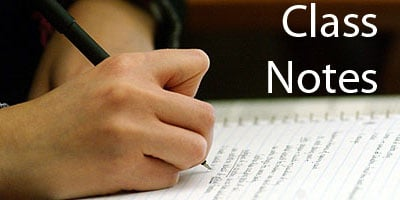## Maths Class 9 Notes for Probability

MEANING OF PROBABILITY

In everyday life, we come across statements such as :

1. It will probably rain today.

2. I doubt that he will pass the test.

3. Most probably, Kavita will stand first in the annual examination.

4. Chances are high that the prices of diesel will go Up.

5. There is a 50-50 chance of India winning a toss in today’s match.

The words ‘probably’, ‘doubt’, ‘most probably’, ‘chances’, etc. used in the statements above involve an element of uncertainty. For example, in (1), ‘probably rain’ will mean it may rain or may not rain today. We are predicting rain today based on our past experience when it rained under similar conditions. Similar predictions are
also made in other cases listed in (2) to (5).

The uncertainty of ‘probably’ etc. can be measured numerically be means of `probability’ in many cases.

SOME IMPORTANT OBJECTS

(i) Coin : We know that a coin has two faces : They are called Head and Tail.(ii) Die : (Dice) : Die is a solid in the form of a cube, having six faces.Each face has some marking of dots as shown above.

One face has one dot, second face two dots, third face has three dots and … so on. We take them as 1, 2, 3, 4, 5, 6. Plural of die is dice.

(iii) Cards : A pack of cards has 52 cards out of which 26 are red cards and 26 are black cards.

(a) 26 red cards contain 13 cards of diamond (♦) and 13 cards of heart (♥).

(b) 26 black cards contain 13 cards spade (♠) and 13 cards of club (♣)

(c) 13 cards are 1, 2, 3, …, 10, Jack, Queen and King.

(d) Card having 1 is also called an ace.

PROBABILITY — AN EXPERIMENTAL (EMPIRICAL) APPROACH.

A trial is an action which results in one or several outcomes. An event for an experiment is the collection of some outcomes of the experiment.

Let n be the total number of trials. The empirical probability P(E) of an event E happening, is given byNumber of trials in which the event happened

Note : The empirical probability depends on the number of trials.

SOME BASIC TERMS AND CONCEPTS:

1. An Experiment : An action or operation resulting in two or more outcomes is called an experiment

EX. (i) Tossing of a coin is an experiment.

There are two possible outcomes head or tail

(ii) Drawing a card from a pack of 52 cards is an experiment There are 52 possible outcomes.

2. Sample space : The set of all possible outcomes of an experiment is called the sample space, denoted by S. An element of S is called a sample point.

Ex. (i) In the experiment of tossing of a coin, the sample space has two points corresponding to head (H) and Tail (T) i.e S {H,T}.

Ex. (ii) When we throw a die then any one of the numbers 1, 2, 3, 4, 5 and 6 will come up. So the sample space, S = {1, 2, 3, 4, 5, 6}

3. Event: Any subset of sample space is an event.

Ex. (i) If the experiment is done throwing a die which has faces numbered 1 to 6, then S= {1,2,3,4, 5,6},A= {1,3,5},B {2, 4, 6}, the null set Φ and S itself are some events with respect to S.The null set Φ is called the impossible event or null event.

(ii) Getting 7 when a die is thrown is called a null event.

The entire sample space is called the certain event.

Some Notes :

(a) The probability of an event lies between 0 and 1, i.e., It can be any fraction from 0 to 1.

(b) The sum of the probabilities of all the possible outcomes of a trial is 1.

(c) Probability of the occurence of an event + Probability of the non-occurrence of that event = 1.